Courses

# Flow Dynamics in Open Channels and Rivers (Part - 3) Civil Engineering (CE) Notes | EduRev

## Civil Engineering (CE) : Flow Dynamics in Open Channels and Rivers (Part - 3) Civil Engineering (CE) Notes | EduRev

The document Flow Dynamics in Open Channels and Rivers (Part - 3) Civil Engineering (CE) Notes | EduRev is a part of Civil Engineering (CE) category.
All you need of Civil Engineering (CE) at this link: Civil Engineering (CE)

In many flow problems it is enough to make a qualitative sketch of water surface profile for a given flow that is taking place between two locations. It is not necessary therefore to find out the exact level of water at different points but the general shape of the free surface has to be drawn as accurately as possible. An analysis of water surface profile may be done by studying the governing equation, which can be derived from the sketch in Figure 20.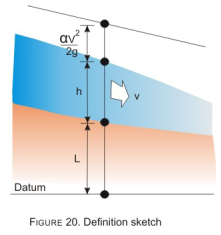Figure 20. Definition sketch

The total energy H at a channel section is given as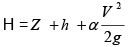(37)

Where

• H: Elevation of energy line above the datum
• Z: Elevation of channel bottom above datum
• h: Flow depth
• V: Mean flow velocity
• α : Velocity head coefficient

Considering x as the space coordinate, taken positive in the direction of flow one obtains by differentiating both sides of the equation (36) with respect to x and expressing V in terms of discharge Q.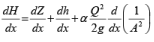(38)

Again, we know by definition:

dH/dx = - Sf                      (39)

And dZ/dx = -S0                 (40)

In which

• Sf: Slope of the energy grade line
• So: Slope of the channel bottom.

The negative sign of Sf and So indicates that both H and Z decrease as x increases. In equation (37) an expression for the derivative of A-2 may be found out as follows: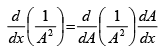(41)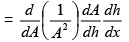(42)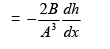(43)

Since dA/dh =B

By substituting equations (39), (40) and (43) into equation (38), and rearranging the resulting equation one obtains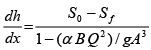(44)

If the channel is not prismatic, then the cross sectional area A changes with distance, and may be expressed as: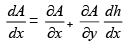(45)

The above change would modify equations (40) and (43) accordingly.

We may express equation (43), which describes the variation of h with x, in terms of the Froude Number (Fr) if we note the following: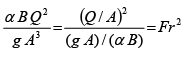(46)

Hence, equation (39) may be written as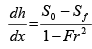(47)

Equation (47) can give a general idea about the nature of the curve if one knows the relative inclinations of the channel bed slope and friction slope (Sf) and the Froude Number (Fr). This may be done by observing the water flow depth (h) with respect to normal depth (hn) and critical depth (hc) for a given discharge, the following figures show the relative changes of hn and hc as channel bed slope is increased gradually from horizontal. It may be observed that the for a given discharge hc does not change but hn goes on decreasing starting from an infinite value for a flat slope.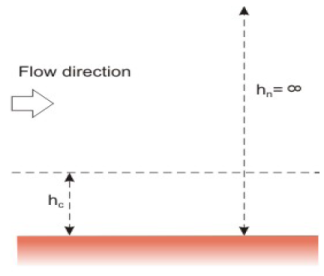Figure 21. Normal depth (hn) and critical depth (hc) on horizontal sloped bed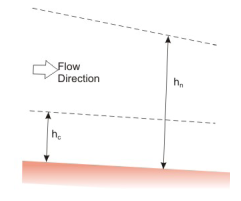Figure 22. Slope increased slightly h> hc; mild slope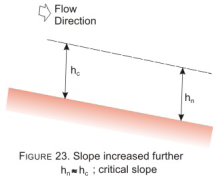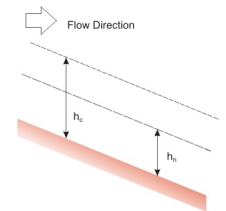Figure 24. Slope increased substantially hn < hc; Steep slope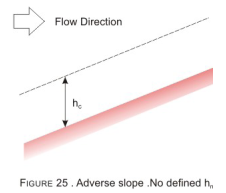In water resources projects, one generally encounters slopes of channels that are either of the following:

• Mild, w here hn>hc                                  (Figure 22)
• Steep, w here hn <hc                         (Figure 24)
• Critical, w here hn = hc                       (Figure 23)
• Flat, w here hn = ∞                              (Figure 21)
• Adverse, where the slope is reversed  (Figure 25)

For each of these slopes, the actual water surface would vary depending upon a control that exist either at the upstream or downstream end of the channel. Some examples of controls are given below

• Weir or spillway   (Figures 26 and 27)
• Gate                    (Figure 28)
• Free overfa ll       (Figure 29)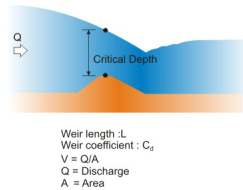Figure 26. Flow over a weir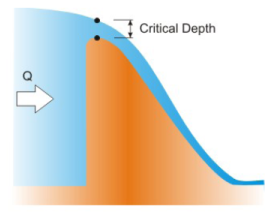Spillway Length : L

V = Q/A

Q = Discharge

A - Area

Figure 27. Flow over a dam spillway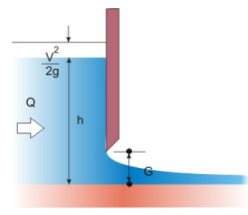Figure 28. Flow below a gate The depth of water just upstream of the gate (h) may be determined from the formula Q=C.LG.VTl where H=h+v2/2g. The formula is valid for small gate openings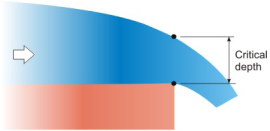Figure 29. Free over fall

Apart from the above a normal depth may be assumed to exist within a very long channel, for which the conditions at the far end may be neglected (Figure 30).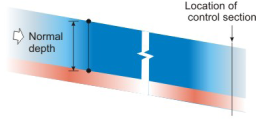Figure 30. Flow in a long channel

The situation shown in Figure 30 is used often while analyzing flow in, say, at the tail end of long irrigation channels or in a long river. Examples illustrating the use of equation (42) and a known control section in determining flow profiles where for a mildly slope channel. Similar profiles may be qualitatively sketched for other channels too.

Offer running on EduRev: Apply code STAYHOME200 to get INR 200 off on our premium plan EduRev Infinity!

,

,

,

,

,

,

,

,

,

,

,

,

,

,

,

,

,

,

,

,

,

;